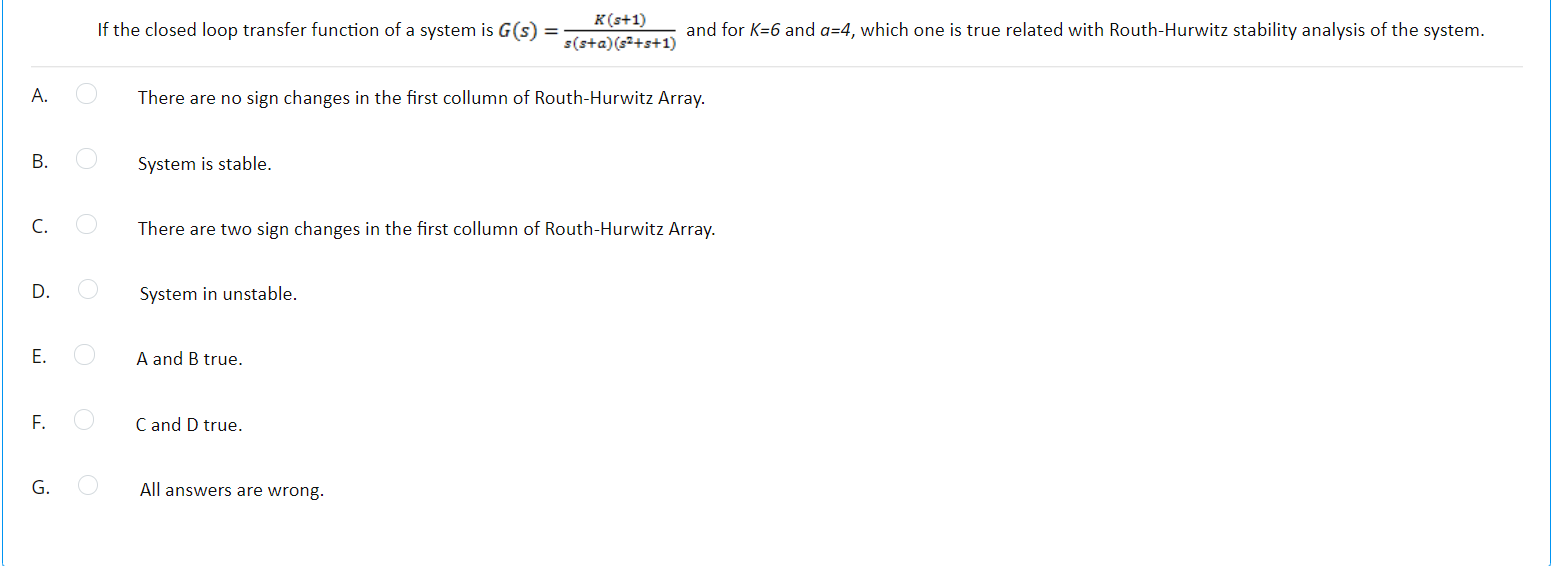# Question If the closed loop transfer function of a system is G(s) K(s+1) s(s+a) (2+s+1) and for K=6 and a=4, which one is true related with Routh-Hurwitz stability analysis of the system. A. There are no sign changes in the first collumn of Routh-Hurwitz Array. B. System is stable. C. There are two sign changes in the first collumn of Routh-Hurwitz Array. D. System in unstable. E. A and B true. F. Cand D true. G. All answers are wrong.WROYIM The Asker · Electrical EngineeringTranscribed Image Text: If the closed loop transfer function of a system is G(s) K(s+1) s(s+a) (2+s+1) and for K=6 and a=4, which one is true related with Routh-Hurwitz stability analysis of the system. A. There are no sign changes in the first collumn of Routh-Hurwitz Array. B. System is stable. C. There are two sign changes in the first collumn of Routh-Hurwitz Array. D. System in unstable. E. A and B true. F. Cand D true. G. All answers are wrong.
More
Transcribed Image Text: If the closed loop transfer function of a system is G(s) K(s+1) s(s+a) (2+s+1) and for K=6 and a=4, which one is true related with Routh-Hurwitz stability analysis of the system. A. There are no sign changes in the first collumn of Routh-Hurwitz Array. B. System is stable. C. There are two sign changes in the first collumn of Routh-Hurwitz Array. D. System in unstable. E. A and B true. F. Cand D true. G. All answers are wrong.